Next: Scalar Triple Product Up: Vector Algebra and Vector Previous: Vector Product

Rotation

Let us try to define a rotation vectorwhose magnitude is the angle of the rotation,, and whose direction is parallel to the axis of rotation, in the sense determined by a right-hand circulation rule. Unfortunately, this is not a good vector. The problem is that the addition of rotations is not commutative, whereas vector addition is commuative. Figure A.105 shows the effect of applying two successive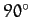rotations, one about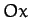, and the other about the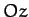, to a standard six-sided die. In the left-hand case, the-rotation is applied before the-rotation, and vice versa in the right-hand case. It can be seen that the die ends up in two completely different states. In other words, the-rotation plus the-rotation does not equal the-rotation plus the-rotation. This non-commuting algebra cannot be represented by vectors. So, although rotations have a well-defined magnitude and direction, they are not vector quantities.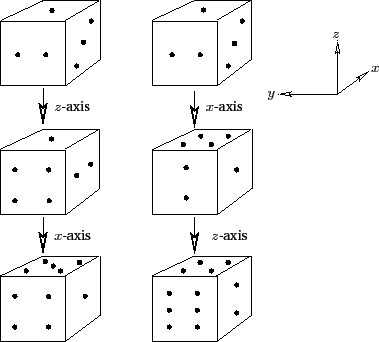But, this is not quite the end of the story. Suppose that we take a general vector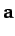and rotate it aboutby a small angle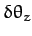. This is equivalent to rotating the coordinate axes about theby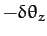. According to Equations (A.1280)-(A.1282), we have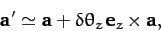(1305)

where use has been made of the small angle approximations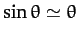and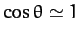. The above equation can easily be generalized to allow small rotations aboutand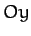by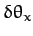and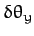, respectively. We find that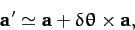(1306)

where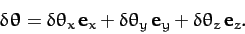(1307)

Clearly, we can define a rotation vector,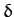, but it only works for small angle rotations (i.e., sufficiently small that the small angle approximations of sine and cosine are good). According to the above equation, a small-rotation plus a small-rotation is (approximately) equal to the two rotations applied in the opposite order. The fact that infinitesimal rotation is a vector implies that angular velocity,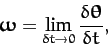(1308)

must be a vector as well. Also, if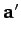is interpreted as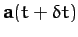in Equation (A.1306) then it follows that the equation of motion of a vector which precesses about the origin with some angular velocity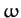is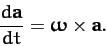(1309)Next: Scalar Triple Product Up: Vector Algebra and Vector Previous: Vector Product
Richard Fitzpatrick 2011-03-31